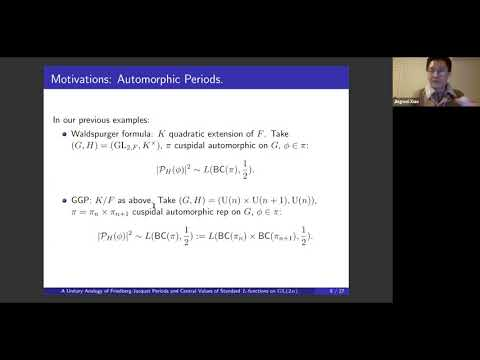## A unitary analogy of Friedberg-Jacquet and Guo-Jacquet periods and central values of standard L functions on GL(2n)

December 3, 2020
IAS
Presenters: Jingwei Xiao

Watch Video

#### Abstract

Let GG be a reductive group over a number field FF and HH a subgroup. Automorphic periods study the integrals of cuspidal automorphic forms on GG over H(F)∖H(AF)H(F)∖H(AF). They are often related to special values of certain L functions. One of the most notable case is when (G,H)=(U(n+1)×U(n),U(n))(G,H)=(U(n+1)×U(n),U(n)), and these periods are related to central values of Rankin-Selberg L functions on GL(n+1)×GL(n)GL(n+1)×GL(n). In this talk, I will explain my work in progress with Wei Zhang that studies central values of standard L functions on GL(2n)GL(2n) using (G,H)=(U(2n),U(n)×U(n))(G,H)=(U(2n),U(n)×U(n)) and some variants. I shall explain the conjecture and a relative trace formula approach to study it. We prove the required fundamental lemma using a limit of the Jacquet-Rallis fundamental lemma and Hironaka’s characterization of spherical functions on the space of non degenerate Hermitian matrices. Also, the question admits an arithmetic analogy.